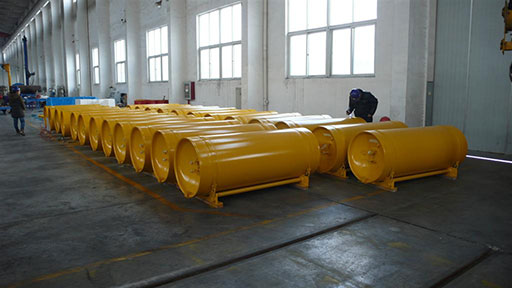# 8.2.4  Carry out a risk assessment and prioritise the risks

Risk is the likelihood of a hazard affecting the water supply system. A risk assessment can be carried out if the risk and the severity of the associated hazard are known. A method of undertaking a risk assessment quantitatively is to use a risk matrix as shown in Figure 8.5 (Bartram et al, 2009). The severity of the impact of a hazard and its likelihood can be multiplied together to arrive at a figure that indicates its risk score. The risk rating will be determined by this risk score, as shown at the bottom of Figure 8.5.

To illustrate this application of a risk matrix, suppose you wanted to carry out a risk assessment for the case where a treatment plant runs out of chlorine disinfectant (Figure 8.6). In a properly managed plant, with a system of stock control in place, such an occurrence may be rare (with a rating of 1). The public health impact of it, however, will be catastrophic (with a rating of 5). Using the matrix in Figure 8.5, you can calculate a risk score, which will be the product of 1 and 5. This risk score of 5 can then be given a risk rating. From the scale in Figure 8.5, this would be ‘low’ (being less than 6).

By repeating the exercise for other hazards and risks and comparing the risk scores, a prioritised list can be drawn up that ranks the risks in order of importance. Unfortunately, budgets are often limited and because of this many of the smaller risks are disregarded.Figure 8.6  Drums of chlorine – essential stocks for a water treatment plant.

8.2.3  Identify the hazards and hazardous events

8.2.5  Identify the control measures needed for each risk